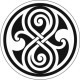# physics4me

physicsgg

## Heisenberg Uncertainty Relation Revisited

with one comment

Kazuo Fujikawa
It is shown that all the known uncertainty relations are the secondary consequences of Robertson’s relation.
The basic idea is to use the Heisenberg picture so that the time development of quantum mechanical operators incorporate the effects of the measurement interaction.
A suitable use of triangle inequalities then gives rise to various forms of uncertainty relations.
The assumptions of unbiased measurement and unbiased disturbance are important to simplify the resulting uncertainty relations and to give the familiar uncertainty relations such as a naive Heisenberg error-disturbance relation.
These simplified uncertainty relations are however valid only conditionally.
Quite independently of uncertainty relations, it is shown that the notion of precise measurement is incompatible with the assumptions of unbiased measurement and unbiased disturbance.
We can thus naturally understand the failure of the naive Heisenberg’s error-disturbance relation, as was demonstrated by the recent spin-measurement by J. Erhart, et al.

Written by physicsgg

December 31, 2013 at 8:50 am

Posted in QUANTUM PHYSICS

### One Response